# Frank solutions for Class 9 Maths ICSE chapter 27 - Trigonometrical Ratios of Standard Angles [Latest edition]

#### Chapters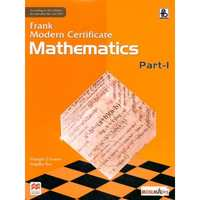## Chapter 27: Trigonometrical Ratios of Standard Angles

Exercise 27.1Exercise 27.2Exercise 27.3
Exercise 27.1

### Frank solutions for Class 9 Maths ICSE Chapter 27 Trigonometrical Ratios of Standard Angles Exercise 27.1

Exercise 27.1 | Q 1.01

Without using tables, evaluate the following: sin60° sin30°+ cos30° cos60°

Exercise 27.1 | Q 1.02

Without using tables, evaluate the following: sec30° cosec60° + cos60° sin30°.

Exercise 27.1 | Q 1.03

Without using tables, evaluate the following sec45° sin45° - sin30° sec60°.

Exercise 27.1 | Q 1.04

Without using tables, evaluate the following: sin230° sin245° + sin260° sin290°.

Exercise 27.1 | Q 1.05

Without using tables, evaluate the following: tan230° + tan260° + tan245°

Exercise 27.1 | Q 1.06

Without using tables, evaluate the following: sin230° cos245° + 4tan230° + sin290° + cos2

Exercise 27.1 | Q 1.07

Without using tables, evaluate the following: cosec245° sec230° - sin230° - 4cot245° + sec260°.

Exercise 27.1 | Q 1.08

Without using tables, evaluate the following: cosec330° cos60° tan345° sin290° sec245° cot30°.

Exercise 27.1 | Q 1.09

Without using tables, evaluate the following: (sin90° + sin45° + sin30°)(sin90° - cos45° + cos60°).

Exercise 27.1 | Q 1.1

Without using tables, evaluate the following: 4(sin430° + cos460°) - 3(cos245° - sin290°).

Exercise 27.1 | Q 2.1

Without using tables, find the value of the following: (sin30° - sin9° + 2cos0°)/(tan30° tan60°)

Exercise 27.1 | Q 2.2

Without using tables, find the value of the following: (sin30°)/(sin45°) + (tan45°)/(sec60°) - (sin60°)/(cot45°) - (cos30°)/(sin90°)

Exercise 27.1 | Q 2.3

Without using tables, find the value of the following: (tan45°)/("cosec"30°) + (sec60°)/(cot45°) - (5sin90°)/(2cos0°)

Exercise 27.1 | Q 2.4

Without using table, find the value of the following: (tan^2 60° + 4cos^2 45° + 3sec^2 30° + 5cos90°)/(cosec30° + sec60° - cot^2 30°)

Exercise 27.1 | Q 2.5

Without using tables, find the value of the following: (4)/(cot^2 30°) + (1)/(sin^2 60°) - cos^2 45°

Exercise 27.1 | Q 3.1

Prove that: sin60°. cos30° - sin60°. sin30° = (1)/(2)

Exercise 27.1 | Q 3.2

Prove that : cos60° . cos30° - sin60° . sin30° = 0

Exercise 27.1 | Q 3.3

Prove that : sec245° - tan245° = 1

Exercise 27.1 | Q 3.4

Prove that: ((cot30° + 1)/(cot30° -1))^2 = (sec30° + 1)/(sec30° - 1)

Exercise 27.1 | Q 4.1

Find the value of 'A', if 2 cos A = 1

Exercise 27.1 | Q 4.2

Find the value of 'A', if 2 sin 2A = 1

Exercise 27.1 | Q 4.3

Find the value of 'A', if cosec 3A = (2)/sqrt(3)

Exercise 27.1 | Q 4.4

Find the value of 'A', if 2cos 3A = 1

Exercise 27.1 | Q 4.5

Find the value of 'A', if sqrt(3)cot"A" = 1

Exercise 27.1 | Q 4.6

Find the value of 'A', if cot 3A = 1

Exercise 27.1 | Q 5.1

Find the value of 'A', if (1 - cosec A)(2 - sec A) = 0

Exercise 27.1 | Q 5.2

Find the value of 'A', if (2 - cosec 2A) cos 3A = 0

Exercise 27.1 | Q 6

If sin α + cosβ = 1 and α= 90°, find the value of 'β'.

Exercise 27.1 | Q 7.1

Solve for 'θ': sin  θ/(3) = 1

Exercise 27.1 | Q 7.2

Solve for 'θ': cot2(θ - 5)° = 3

Exercise 27.1 | Q 7.3

Solve for 'θ': sec(θ/2 + 10°) = (2)/sqrt(3)

Exercise 27.1 | Q 8.1

Find the value of x in the following:  2 sin3x = sqrt(3)

Exercise 27.1 | Q 8.2

Find the value of x in the following: 2sin  x/(2) = 1

Exercise 27.1 | Q 8.3

Find the value of x in the following: sqrt(3)sin x = cos x

Exercise 27.1 | Q 8.4

Find the value of x in the following: tan x = sin45° cos45° + sin30°

Exercise 27.1 | Q 8.5

Find the value of x in the following: sqrt(3)tan 2x = cos60° + sin45° cos45°

Exercise 27.1 | Q 8.6

Find the value of x in the following: cos2x = cos60° cos30° + sin60° sin30°

Exercise 27.1 | Q 9

If sinθ = cosθ and 0° < θ<90°, find the value of 'θ'.

Exercise 27.1 | Q 10

If tanθ= cotθ and 0°≤ θ ≤ 90°, find the value of 'θ'.

Exercise 27.1 | Q 11

If sqrt(2) = 1.414 and sqrt(3) = 1.732, find the value of the following correct to two decimal places tan60°

Exercise 27.1 | Q 12.1

If θ = 30°, verify that: tan2θ = (2tanθ)/(1 - tan^2θ)

Exercise 27.1 | Q 12.2

If θ = 30°, verify that: sin2θ = (2tanθ)/(1 ++ tan^2θ)

Exercise 27.1 | Q 12.3

If A = 30°, verify that cos2θ = (1 - tan^2 θ)/(1 + tan^2 θ) = cos4θ - sin4θ = 2cos2θ - 1 - 2sin2θ

Exercise 27.1 | Q 12.4

If θ = 30°, verify that: sin 3θ = 4sinθ . sin(60° - θ) sin(60° + θ)

Exercise 27.1 | Q 12.5

If θ = 30°, verify that: 1 - sin 2θ = (sinθ - cosθ)2

Exercise 27.1 | Q 13.1

Evaluate the following: ((sin3θ - 2sin4θ))/((cos3θ - 2cos4θ)) when 2θ = 30°

Exercise 27.1 | Q 13.2

Evaluate the following: ((1 - cosθ)(1 + cosθ))/((1 - sinθ)(1 + sinθ) if θ = 30°

Exercise 27.1 | Q 14

If θ = 15°, find the value of: cos3θ - sin6θ + 3sin(5θ + 15°) - 2 tan2

Exercise 27.1 | Q 15.1

If A = B = 60°, verify that: cos(A - B) = cosA cosB + sinA sinB

Exercise 27.1 | Q 15.2

If A = B = 60°, verify that: sin(A - B) = sinA cosB - cosA sinB

Exercise 27.1 | Q 15.3

If A = B = 60°, verify that: tan(A - B) = (tan"A" - tan"B")/(1 + tan"A" tan"B"")

Exercise 27.1 | Q 16.1

If A = 30° and B = 60°, verify that: sin (A + B) = sin A cos B + cos A sin B

Exercise 27.1 | Q 16.2

If A = 30° and B = 60°, verify that: cos (A + B) = cos A cos B - sin A sin B

Exercise 27.1 | Q 16.3

If A = 30° and B = 60°, verify that: (sin("A" + "B"))/(cos"A" . cos"B") = tanA + tanB

Exercise 27.1 | Q 16.4

If A = 30° and B = 60°, verify that: (sin("A" -"B"))/(sin"A" . sin"B") = cotB - cotA

Exercise 27.1 | Q 17.1

If A = B = 45°, verify that sin (A - B) = sin A .cos B - cos A.sin B

Exercise 27.1 | Q 17.2

If A = B = 45°, verify that cos (A - B) = cosA.cos B + sin A.sin B

Exercise 27.1 | Q 18

If sin(A - B) = sinA cosB - cosA sinB and cos(A - B) = cosA cosB + sinA sinB, find the values of sin15° and cos15°.

Exercise 27.1 | Q 19.1

If θ < 90°, find the value of: sin2θ + cos2θ

Exercise 27.1 | Q 19.2

If θ < 90°, find the value of: tan^2θ - (1)/cos^2θ

Exercise 27.1 | Q 20.1

If sqrt(3)sec 2θ = 2 and θ< 90°, find the value of θ

Exercise 27.1 | Q 20.2

If sqrt(3) sec 2θ = 2 and θ< 90°, find the value of

cos 3θ

Exercise 27.1 | Q 20.3

If sqrt(3) sec 2θ = 2 and θ< 90°, find the value of

cos2 (30° + θ) + sin2 (45° - θ)

Exercise 27.1 | Q 21

In the given figure, PQ = 6 cm, RQ = x cm and RP = 10 cm, find
a. cosθ
b. sin2θ- cos2θ
c. Use tanθ to find the value of RQ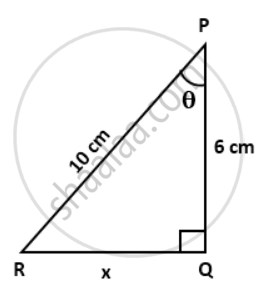Exercise 27.1 | Q 22

Find the value of: sqrt((1 - sin^2 60°)/(1 + sin^2 60°) If 3 tan2θ - 1 = 0, find the value
a. cosθ
b. sinθ

Exercise 27.1 | Q 23

If sin(A +B) = 1(A -B) = 1, find A and B.

Exercise 27.1 | Q 24

If tan(A - B) = (1)/sqrt(3) and tan(A + B) = sqrt(3), find A and B.

Exercise 27.1 | Q 25

If sin(A - B) = (1)/(2) and cos(A + B) = (1)/(2), find A and B.

Exercise 27.1 | Q 26

In ΔABC right angled at B, ∠A = ∠C. Find the value of:
(i) sinA cosC + cosA sinC
(ii) sinA sinB + cosA cosB

Exercise 27.1 | Q 27

If tan "A" = (1)/(2), tan "B" = (1)/(3) and tan("A" + "B") = (tan"A" + tan"B")/(1 - tan"A" tan"B"), find A + B.

Exercise 27.2

### Frank solutions for Class 9 Maths ICSE Chapter 27 Trigonometrical Ratios of Standard Angles Exercise 27.2

Exercise 27.2 | Q 1.1

Find the value of 'x' in each of the following: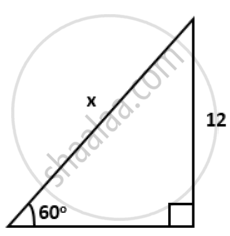Exercise 27.2 | Q 1.2

Find the value of 'x' in each of the following: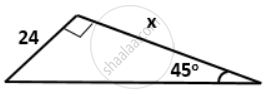Exercise 27.2 | Q 1.3

Find the value of 'x' in each of the following: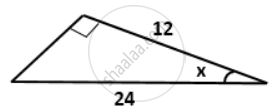Exercise 27.2 | Q 1.4

Find the value of 'x' in each of the following: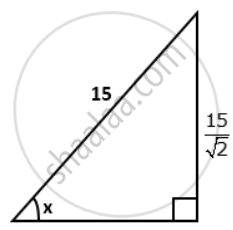Exercise 27.2 | Q 2

Find the length of AD. Given: ∠ABC = 60°, ∠DBC = 45° and BC = 24 cm.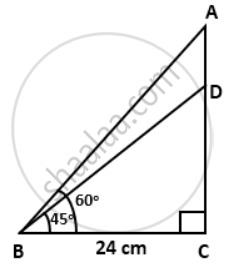Exercise 27.2 | Q 3

Find lengths of diagonals AC and BD. Given AB = 24 cm and ∠BAD = 60°.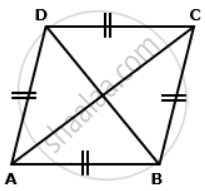Exercise 27.2 | Q 4

In a trapezium ABCD, as shown, AB ‖ DC, AD = DC = BC = 24 cm and ∠A = 30°. Find: length of AB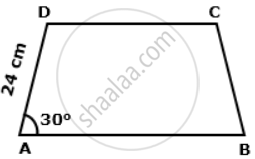Exercise 27.2 | Q 5

Find the length of EC.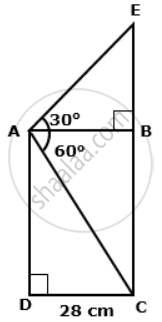Exercise 27.2 | Q 6

In the given figure, AB and EC are parallel to each other. Sides AD and BC are 1.5 cm each and are perpendicular to AB. Given that ∠AED = 45° and ∠ACD = 30°. Find:
a. AB
b. AC
c. AE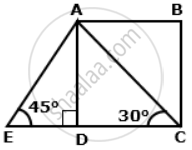Exercise 27.2 | Q 7

In the given figure, ∠B = 60°, ∠C = 30°, AB = 8 cm and BC = 24 cm. Find:
a. BE
b. AC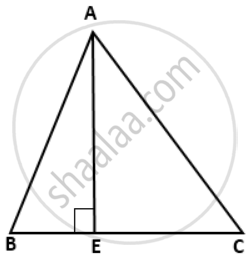Exercise 27.2 | Q 8

Find:
a. BC
c. AC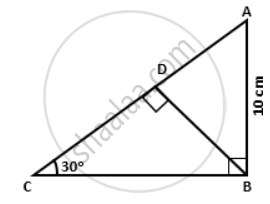Exercise 27.2 | Q 9.1

Find the value 'x', if: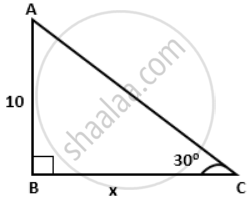Exercise 27.2 | Q 9.2

Find the value 'x', if: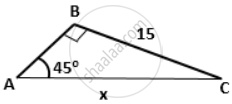Exercise 27.2 | Q 9.3

Find the value 'x', if: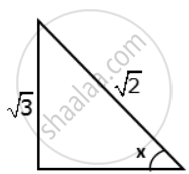Exercise 27.2 | Q 9.4

Find the value 'x', if: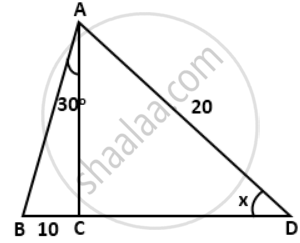Exercise 27.2 | Q 9.5

Find the value 'x', if: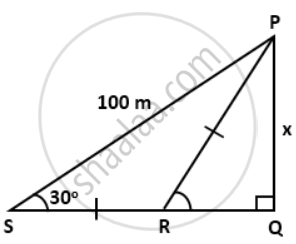Exercise 27.2 | Q 9.6

Find the value 'x', if: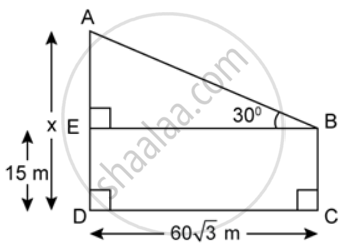Exercise 27.2 | Q 10.1

Find the value of 'y' if sqrt(3) = 1.723.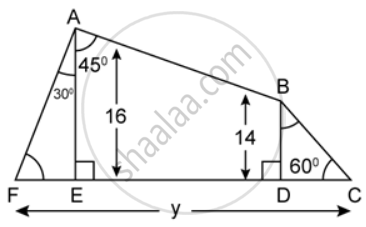Exercise 27.2 | Q 10.2

Find the value of 'y' if sqrt(3) = 1.723.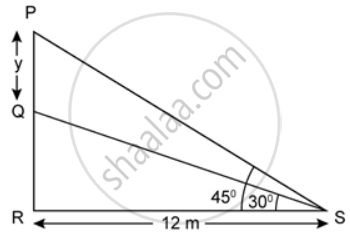Exercise 27.2 | Q 11

In the given figure, if tan θ = (5)/(13), tan  α = (3)/(5) and RS = 12m, find the value of 'h'.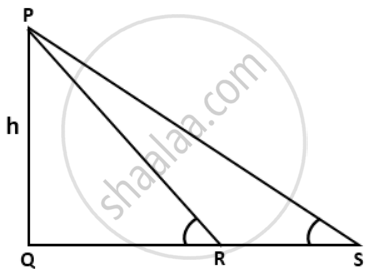Exercise 27.2 | Q 12.1

Find x and y, in each of the following figure: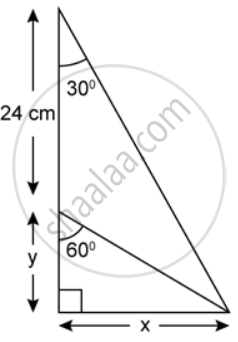Exercise 27.2 | Q 12.2

Find x and y, in each of the following figure: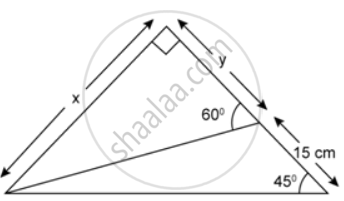Exercise 27.2 | Q 13

If tan x° = (5)/(12) . tan y° = (3)/(4) and AB = 48m; find the length CD.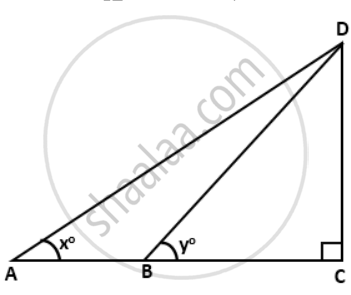Exercise 27.2 | Q 14

In a right triangle ABC, right angled at C, if ∠B = 60° and AB = 15units, find the remaining angles and sides.

Exercise 27.2 | Q 15

If ΔABC is a right triangle such that ∠C = 90°, ∠A = 45° and BC =7units, find ∠B, AB and AC.

Exercise 27.2 | Q 16

In a rectangle ABCD, AB = 20cm, ∠BAC = 60°, calculate side BC and diagonals AC and BD.

Exercise 27.2 | Q 17

In right-angled triangle ABC; ∠B = 90°. Find the magnitude of angle A, if:
a. AB is sqrt(3) times of BC.
B. BC is sqrt(3) times of BC.

Exercise 27.2 | Q 18

A ladder is placed against a vertical tower. If the ladder makes an angle of 30° with the ground and reaches upto a height of 18 m of the tower; find length of the ladder.

Exercise 27.2 | Q 19

The perimeter of a rhombus is 100 cm and obtuse angle of it is 120°. Find the lengths of its diagonals.

Exercise 27.2 | Q 20

In the given figure; ∠B = 90°, ∠ADB = 30°, ∠ACB = 45° and AB = 24 m. Find the length of CD.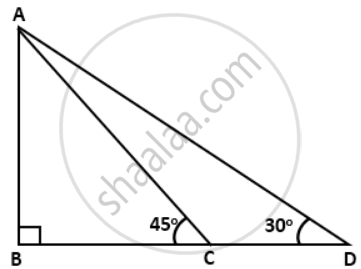Exercise 27.2 | Q 21

In the given figure, a rocket is fired vertically upwards from its launching pad P. It first rises 20 km vertically upwards and then 20 km at 60° to the vertical. PQ represents the first stage of the journey and QR the second. S is a point vertically below R on the horizontal level as P, find:
a. the height of the rocket when it is at point R.
b. the horizontal distance of point S from P.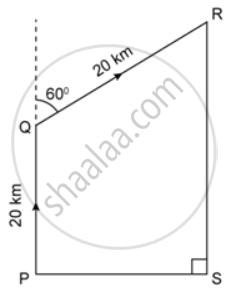Exercise 27.3

### Frank solutions for Class 9 Maths ICSE Chapter 27 Trigonometrical Ratios of Standard Angles Exercise 27.3

Exercise 27.3 | Q 1.1

Evaluate the following: (sin62°)/(cos28°)

Exercise 27.3 | Q 1.2

Evaluate the following: (sec34°)/("cosec"56°)

Exercise 27.3 | Q 1.3

Evaluate the following: (tan12°)/(cot78°)

Exercise 27.3 | Q 1.4

Evaluate the following: (sin25° cos43°)/(sin47° cos 65°)

Exercise 27.3 | Q 1.5

Evaluate the following: (sec32° cot26°)/(tan64° "cosec"58°)

Exercise 27.3 | Q 1.6

Evaluate the following: (cos34° cos35°)/(sin57° sin56°)

Exercise 27.3 | Q 2.1

Evaluate the following: sin31° - cos59°

Exercise 27.3 | Q 2.2

Evaluate the following: cot27° - tan63°

Exercise 27.3 | Q 2.3

Evaluate the following: cosec 54° - sec 36°

Exercise 27.3 | Q 2.4

Evaluate the following: sin28° sec62° + tan49° tan41°

Exercise 27.3 | Q 2.5

Evaluate the following: sec16° tan28° - cot62° cosec74°

Exercise 27.3 | Q 2.6

Evaluate the following: sin22° cos44° - sin46° cos68°

Exercise 27.3 | Q 3.1

Evaluate the following: (sin36°)/(cos54°) + (sec31°)/("cosec"59°)

Exercise 27.3 | Q 3.2

Evaluate the following: (tan42°)/(cot48°) + (cos33°)/(sin57°)

Exercise 27.3 | Q 3.3

Evaluate the following: (2sin28°)/(cos62°) + (3cot49°)/(tan41°)

Exercise 27.3 | Q 3.4

Evaluate the following: (5sec68°)/("cosec"22°) + (3sin52° sec38°)/(cot51° cot39°)

Exercise 27.3 | Q 4.1

Express each of the following in terms of trigonometric ratios of angles between 0° and 45°: sin65° + cot59°

Exercise 27.3 | Q 4.2

Express each of the following in terms of trigonometric ratios of angles between 0° and 45°: cos72° - cos88°

Exercise 27.3 | Q 4.3

Express each of the following in terms of trigonometric ratios of angles between 0° and 45°: cosec64° + sec70°

Exercise 27.3 | Q 4.4

Express each of the following in terms of trigonometric ratios of angles between 0° and 45°: tan77° - cot63° + sin57°

Exercise 27.3 | Q 4.5

Express each of the following in terms of trigonometric ratios of angles between 0° and 45°: sin53° + sec66° - sin50°

Exercise 27.3 | Q 4.6

Express each of the following in terms of trigonometric ratios of angles between 0° and 45°: cos84° + cosec69° - cot68°

Exercise 27.3 | Q 5.01

Evaluate the following: sin35° sin45° sec55° sec45°

Exercise 27.3 | Q 5.02

Evaluate the following: cot20° cot40° cot45° cot50° cot70°

Exercise 27.3 | Q 5.03

Evaluate the following: cos39° cos48° cos60° cosec42° cosec51°

Exercise 27.3 | Q 5.04

Evaluate the following: sin(35° + θ) - cos(55° - θ) - tan(42° + θ) + cot(48° - θ)

Exercise 27.3 | Q 5.05

Evaluate the following: tan(78° + θ) + cosec(42° + θ) - cot(12° - θ) - sec(48° - θ)

Exercise 27.3 | Q 5.06

Evaluate the following: (3sin37°)/(cos53°) - (5"cosec"39°)/(sec51°) + (4tan23° tan37° tan67° tan53°)/(cos17° cos67° "cosec"73° "cosec"23°)

Exercise 27.3 | Q 5.07

Evaluate the following: (sin0° sin35° sin55° sin75°)/(cos22° cos64° cos58° cos90°)

Exercise 27.3 | Q 5.08

Evaluate the following: (2sin25° sin35° sec55° sec65°)/(5tan 29° tan45° tan61°) + (3cos20° cos50° cot70° cot40°)/(5tan20° tan50° sin70° sin40°)

Exercise 27.3 | Q 5.09

Evaluate the following: (3sin^2 40°)/(4cos^2 50°) - ("cosec"^2 28°)/(4sec^2 62°) + (cos10° cos25° cos45° "cosec"80°)/(2sin15° sin25° sin45° sin65° sec75°)

Exercise 27.3 | Q 5.1

Evaluate the following: (5cot5° cot15° cot25° cot35° cot45°)/(7tan45° tan55° tan65° tan75° tan85°) + (2"cosec"12° "cosec"24° cos78° cos66°)/(7sin14° sin23° sec76° sec67°)

Exercise 27.3 | Q 6

If cos3θ = sin(θ - 34°), find the value of θ if 3θ is an acute angle.

Exercise 27.3 | Q 7

If tan4θ = cot(θ + 20°), find the value of θ if 4θ is an acute angle.

Exercise 27.3 | Q 8

If sec2θ = cosec3θ, find the value of θ if it is known that both 2θ and 3θ are acute angles.

Exercise 27.3 | Q 9

If sin(θ - 15°) = cos(θ - 25°), find the value of θ if (θ-15°) and (θ - 25°) are acute angles.

Exercise 27.3 | Q 10

If A, B and C are interior angles of ΔABC, prove that sin(("A" + "B")/2) = cos  "C"/(2)

Exercise 27.3 | Q 11

If P, Q and R are the interior angles of ΔPQR, prove that cot(("Q" + "R")/2) = tan  "P"/(2)

Exercise 27.3 | Q 12

If cosθ = sin60° and θ is an acute angle find the value of 1- 2 sin2θ

Exercise 27.3 | Q 13

If secθ= cosec30° and θ is an acute angle, find the value of 4 sin2θ - 2 cos2θ.

Exercise 27.3 | Q 14.1

Prove the following: tanθ tan(90° - θ) = cotθ cot(90° - θ)

Exercise 27.3 | Q 14.2

Prove the following: sin58° sec32° + cos58° cosec32° = 2

Exercise 27.3 | Q 14.3

Prove the following: (tan(90° - θ)cotθ)/("cosec"^2 θ) = cos2θ

Exercise 27.3 | Q 14.4

Prove the following: sin230° + cos230° = (1)/(2)sec60°

Exercise 27.3 | Q 15

If A + B = 90°, prove that (tan"A" tan"B" + tan"A" cot"B")/(sin"A" sec"B") - (sin^2"B")/(cos^2"A") = tan2A

## Chapter 27: Trigonometrical Ratios of Standard Angles

Exercise 27.1Exercise 27.2Exercise 27.3## Frank solutions for Class 9 Maths ICSE chapter 27 - Trigonometrical Ratios of Standard Angles

Frank solutions for Class 9 Maths ICSE chapter 27 (Trigonometrical Ratios of Standard Angles) include all questions with solution and detail explanation. This will clear students doubts about any question and improve application skills while preparing for board exams. The detailed, step-by-step solutions will help you understand the concepts better and clear your confusions, if any. Shaalaa.com has the CISCE Class 9 Maths ICSE solutions in a manner that help students grasp basic concepts better and faster.

Further, we at Shaalaa.com provide such solutions so that students can prepare for written exams. Frank textbook solutions can be a core help for self-study and acts as a perfect self-help guidance for students.

Concepts covered in Class 9 Maths ICSE chapter 27 Trigonometrical Ratios of Standard Angles are Trigonometric Equation Problem and Solution, Trigonometric Ratios of Some Special Angles, Trigonometric Ratios of Some Special Angles, Trigonometric Ratios of Some Special Angles.

Using Frank Class 9 solutions Trigonometrical Ratios of Standard Angles exercise by students are an easy way to prepare for the exams, as they involve solutions arranged chapter-wise also page wise. The questions involved in Frank Solutions are important questions that can be asked in the final exam. Maximum students of CISCE Class 9 prefer Frank Textbook Solutions to score more in exam.

Get the free view of chapter 27 Trigonometrical Ratios of Standard Angles Class 9 extra questions for Class 9 Maths ICSE and can use Shaalaa.com to keep it handy for your exam preparation